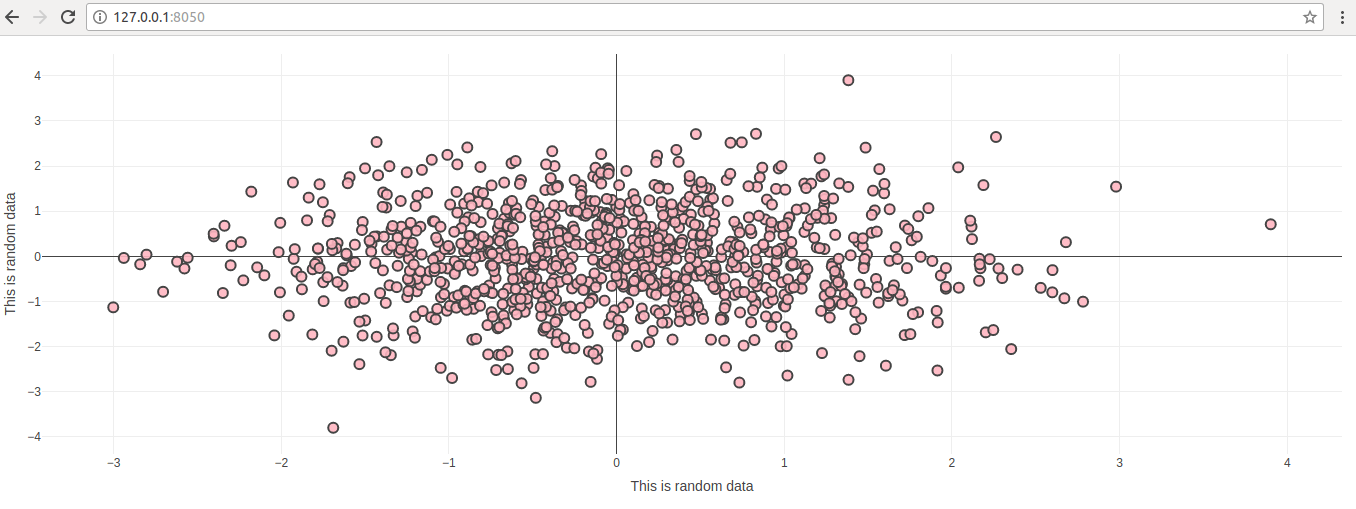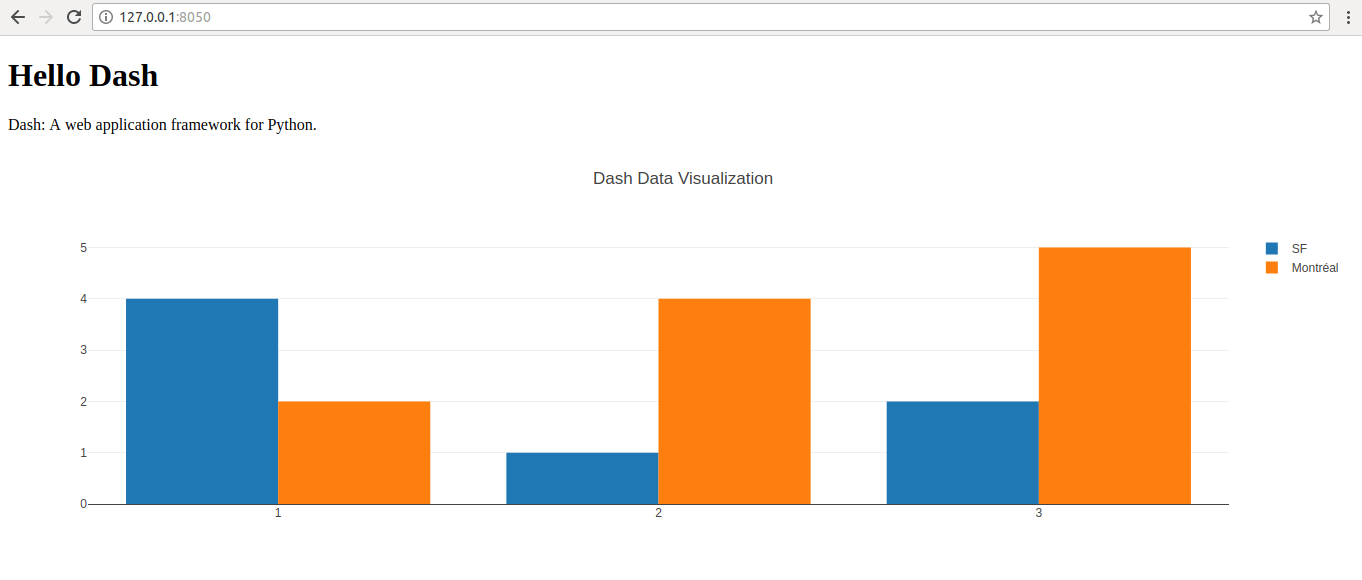Plotly Dash folder Structure for Multi Page application

Normally whenever we present data, we need following two things

1. Interactivity
2. Different view of same or related data

So far we have looked at creating simple single page single graph applications. If you want to show multiple graphs on the same page, you can use multiple dcc.graph function. However, to make applicationmore userfriendly, providing different views at different pages which has different url is more user freindly. Please find below folder structure for creating some application.

├── app.py
├── index.py
└── apps
├── barchart.py
├── commonmodules.py
├── home.py
├── linechart.py
└── scatterplot.py

You can find the code for this boiler plate here

Customize Graphs Using Dash

In this article we will look at different ways of customizing of dash graphs. We will take an example of scatter plot. We will start with simple scatter plot and then build on top of it. Let us start with basic scatter plot which was referred in post Simple graphs using Dash

Simple Scatter plot

import dash
import dash_core_components as dcc
import dash_html_components as html
import pandas as pd
import plotly.graph_objs as go

app = dash.Dash()

import numpy as np

N = 1000
random_x = np.random.randn(N)
random_y = np.random.randn(N)
random_x2 = np.random.randn(N)
random_y2 = np.random.randn(N)

app.layout = html.Div([
dcc.Graph(
id='life-exp-vs-gdp',
figure={
'data': [
go.Scatter(
x = random_x,
y = random_y,
mode = 'markers',

)
],
}
)
])

if __name__ == '__main__':
app.run_server()

OutputNow let us add axis details and change color scheme of these dots on the scatter plot

import dash
import dash_core_components as dcc
import dash_html_components as html
import pandas as pd
import plotly.graph_objs as go

app = dash.Dash()

import numpy as np

N = 1000
random_x = np.random.randn(N)
random_y = np.random.randn(N)
random_x2 = np.random.randn(N)
random_y2 = np.random.randn(N)

app.layout = html.Div([
dcc.Graph(
id='life-exp-vs-gdp',
figure={
'data': [
go.Scatter(
x = random_x,
y = random_y,
mode = 'markers',
marker = dict(
size = 10,
color = 'rgba(255, 182, 193, .9)',
line = dict(
width = 2,
)
)
)
],
'layout': go.Layout(
xaxis={'title': 'This is random data'},
yaxis={'title': 'This is random data'},
margin={'l': 40, 'b': 40, 't': 10, 'r': 10},
legend={'x': 0, 'y': 1},
hovermode='closest'
)
}
)
])

if __name__ == '__main__':
app.run_server()

OutputScatter plot with multiple data sets

Here we have only one data set. Let us see how to add multiple data sets on the same scatter plot

import dash
import dash_core_components as dcc
import dash_html_components as html
import pandas as pd
import plotly.graph_objs as go

app = dash.Dash()

import numpy as np

N = 1000
random_x = np.random.randn(N)
random_y = np.random.randn(N)

app.layout = html.Div([
dcc.Graph(
id='life-exp-vs-gdp',
figure={
'data': [
go.Scatter(
x = random_x,
y = random_y,
mode = 'markers',
marker = dict(
size = 10,
color = 'rgba(152, 0, 0, .8)',
line = dict(
width = 2,
)
)
),
go.Scatter(
x = random_x,
y = random_y+5,
mode = 'markers',
marker = dict(
size = 10,
color = 'rgba(255, 182, 193, .9)',
line = dict(
width = 2,
)
)
)
],
'layout': go.Layout(
xaxis={'title': 'This is random data'},
yaxis={'title': 'This is random data'},
margin={'l': 40, 'b': 40, 't': 10, 'r': 10},
legend={'x': 0, 'y': 1},
hovermode='closest'
)
}
)
])

if __name__ == '__main__':
app.run_server()

OutputYou can customize this further. Whatever you can do with plotly python module, you can do with Dash

Simple graphs using Dash

In previous post, getting started with Dash we saw how to get simple Dash site up and running. We set up Dash site without any graph, now let us add simple graphs to the side.

Simple Bar Chart with Dash

Following is the code for simple Dash Bar chart site.

# -*- coding: utf-8 -*-
import dash
import dash_core_components as dcc
import dash_html_components as html

app = dash.Dash()

app.layout = html.Div(children=[
html.H1(children='Hello Dash'),

html.Div(children='''
Dash: A web application framework for Python.
'''),

dcc.Graph(
id='example-graph',
figure={
'data': [
{'x': [1, 2, 3], 'y': [4, 1, 2], 'type': 'bar', 'name': 'SF'},
{'x': [1, 2, 3], 'y': [2, 4, 5], 'type': 'bar', 'name': u'Montréal'},
],
'layout': {
'title': 'Dash Data Visualization'
}
}
)
])

if __name__ == '__main__':
app.run_server(debug=True)

Here is the outputIf you compare this code with the previous post code, you will see only one different. We have added “dcc.Graph” component here.

This is where magic happens. You can add multiple dcc.Graph cmponents to display multiple graphs on the same page.

Let us look at simple line graph. Please note the only different between bar chart and line chart.

# -*- coding: utf-8 -*-
import dash
import dash_core_components as dcc
import dash_html_components as html

app = dash.Dash()

app.layout = html.Div(children=[
html.H1(children='Hello Dash'),

html.Div(children='''
Dash: A web application framework for Python.
'''),

dcc.Graph(
id='example-graph',
figure={
'data': [
{'x': [1, 2, 3], 'y': [4, 1, 2], 'type': 'bar', 'name': 'SF'},
{'x': [1, 2, 3], 'y': [2, 4, 5], 'type': 'bar', 'name': u'Montréal'},
],
'layout': {
'title': 'Dash Data Visualization'
}
}
)
])

if __name__ == '__main__':
app.run_server(debug=True)

Here is the outputNow let us look at scatter plot

import dash
import dash_core_components as dcc
import dash_html_components as html
import pandas as pd
import plotly.graph_objs as go

app = dash.Dash()

import numpy as np

N = 1000
random_x = np.random.randn(N)
random_y = np.random.randn(N)
random_x2 = np.random.randn(N)
random_y2 = np.random.randn(N)

app.layout = html.Div([
dcc.Graph(
id='life-exp-vs-gdp',
figure={
'data': [
go.Scatter(
x = random_x,
y = random_y,
mode = 'markers',

)
],
}
)
])

if __name__ == '__main__':
app.run_server()

Output is as belowGetting Started with Dash on Ubuntu

To run Dash we need to install multiple python modules. They have broken Dash into multiple components.

Step#1 Installation

sudo python3.6 -m pip install dash==0.22.0 # The core dash backend
sudo python3.6 -m pip installl dash-renderer==0.13.0 # The dash front-end
sudo python3.6 -m pip install dash-html-components==0.11.0 # HTML components
sudo python3.6 -m pip install dash-core-components==0.26.0 # Supercharged components
sudo python3.6 -m pip install plotly --upgrade # Plotly graphing library used in examples

Step#2 Hello World in Dash

This is simplest Dash site

# -*- coding: utf-8 -*-
import dash
import dash_core_components as dcc
import dash_html_components as html

app = dash.Dash()

app.layout = html.Div(children=[
html.H1(children='Hello World'),

html.Div(children='''
This is simplest "Hello World" by using Dash
'''),
])

if __name__ == '__main__':
app.run_server(debug=True)

Step#3 Running Dash site.

\$python3.6 filename.py

Thi will run the Dash site and you can access it at http://127.0.0.1:8050/

Introducing Dash

Dash is a dream combination of JavaScript and Python. Its like best of both the worlds (I still think so). Reactive-ness of JavaScript framework React and Statistical capabilities of Python modules.

When I was working on react applications, I really liked the virtual DOM element and quick responses of react however  JavaScript lacks the statistical libraries that python has.

When I was learning python front-end frameworks django and flask, they both seems archaic as compared to nodejs. django has steep learning curve as well. Creating charts and having real time update using django channels is quiet complex.

I was not aware of dash but while searching for some chart related updates, I came across dash. I was super excited to learn more about Dash.

This excitement got diminished in next few days due to “different” way to writing html. This is simillar confusion as when I started learning JSX. (Yeah, mankind worked for decaded to put JavaScript out of html and ended by putting html inside JavaScript !). I was about to give up on Dash and think of alternatives such as showing matyplotlib graphs on webpage, explored bokeh and even plot.ly (parent of Dash 🙂 ), however, I decided to stick with Dash for a while to see how things are structured.

Dash documentation is not adequate, however its sufficient for you to get started but you need to go through it couple of time. Don’t go through documentation from start to end like a book but find a problem and try to implement it (well, that is the best way to learn any programming language or framework )

To create a web app with Flask or django, and then combining it with interactive JS frameworks, and then incorporating the interactivity with jquery, ajax, and React would take you quite a bit of time and code and this will make application very complex and hard to maintain, however with Dash, this is very easy.